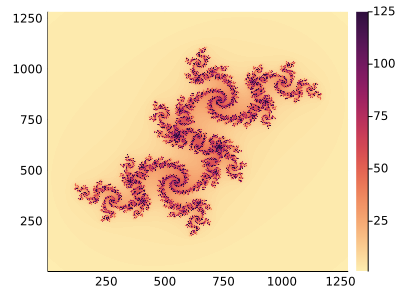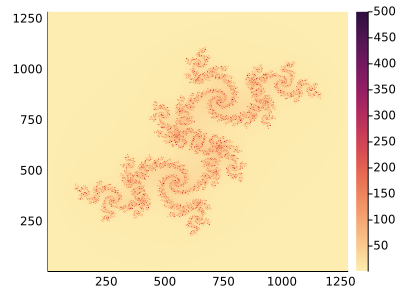# How to Plot Fractals using Plots and GR()?

Hi all,

I have tried this code here:

But I can’t plot it, I am using Jupyter Notebook and Julia REPL it only shows : ` plotJulia`

Did you, er, forget to run the function you’ve defined?1 Like

Wow, so that’s how to plot.

I am just learning Plots and GLMakie, I have no idea till now… it is not forget, but do not know how…

``````function plotJulia(c::Complex{T};
xRng = [-1.5,1.5], yRng = [-1.5, 1.5], maxIters=100) where T<:Real
...
#maxIters =1000  #comment out this line
...
#display(heatmap(iterCounts')) #comment out this line, too
end
``````

and call it as:

``````itc= plotJulia( -0.04 - 0.6841im; maxIters=125)
heatmap(itc',  c = :matter, size=(400, 300))
``````

and then replace `maxIters=125`, by `maxIters=500` and look at colorbars of the two heatmaps. You can deduce that the fractal set corresponds to points that leave the disk of radius 2 in about 100-120 iterations. Hence the number
of iterations must be reduced, because
with 1000 iterations the interval [100, 120] is mapped on lighter colors within the range [1, 1000], and the fractal is poorly visible.
125 iterations:500 iterations:1 Like

That’s amazingly beautiful.

Can we make a video of fractals or zoom in of the fractals?

See a beautiful example here and code here by @federicostra.

1 Like